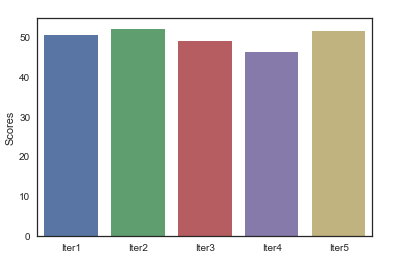# K-Fold Cross Validation Example Using Python scikit-learn

In this post, we will provide an example of Cross Validation using the K-Fold method with the python scikit learn library.  The K-Fold Cross Validation example would have k parameters equal to 5. By using a ‘for’ loop, we will fit each model using 4 folds for training data and 1 fold for testing data, and then we will call the accuracy_score method from scikit learn to determine the accuracy of the model.

The example is divided into the following steps:

• Step 1: Import the libraries and load into the environment Open, High, Low, Close data for EURUSD
• Step 2: Create features with the create_features() function
• Step 3: Run the model with the Validation Set approach
• Step 4: Run the model with the K-Fold Cross Validation approach
import pandas as pd
from sklearn.model_selection import KFold
import numpy as np
from sklearn.metrics import accuracy_score
from sklearn import tree
import matplotlib.pyplot as plt
import seaborn as sns

# Read csv file to load into the environment OHLC data from EURUSD.

# The create_features function, receives the eurusd_ohlc parameter  and create new features to use in a machine learning model

def create_features(fx_data):
'''
Parameters:
fx_data: has Open-High-Low-Close data for currency pair EURUSD between  2001-08-21 to 2019-09-21
Return:
fx_data: dataframe with original and new data with the features of the model
target: target variable to predict, which contains the direction of the price.  The values can be 1 for up direction and -1 for down direction.
'''

# Convert all columns of the stock_data data frame to numeric columns
fx_data = fx_data.apply(pd.to_numeric)

# Reverse the index to have old values at top of the dataframe
fx_data = fx_data.sort_values('Date')

# Create features to use in the machine learning model
fx_data['High-Low'] = fx_data['High'] - fx_data['Low']
fx_data['pct_change'] = fx_data['Close'].pct_change()
fx_data['ret_5'] = fx_data['pct_change'].rolling(5).mean()

# Calculate RSI Indicator
close = fx_data['Close']
# Get the difference in price from previous step
delta = close.diff()
# Get rid of the first row, which is Nan since it did not have a previous
# row to calculate the differences
delta = delta[1:]

# Make the positive gains (up) and negative gains (down) Series
up, down = delta.copy(), delta.copy()
up[up < 0] = 0
down[down > 0] = 0

# Calculate the EWMA
roll_up = up.rolling(center=False,window=14).mean()
roll_down = abs(down).rolling(center=False,window=14).mean()

# Calculate the RSI based on EWMA
RS = roll_up / roll_down
RSI = 100.0 - (100.0 / (1.0 + RS))

fx_data['RSI'] = RSI
fx_data.dropna(inplace=True)
# Create the target variable that take the values of 1 if the stock price go up or -1 if the stock price go down
target = np.where(fx_data['Close'].shift(-1) > fx_data['Close'], 1, -1)

return fx_data, target

features, target = create_features(eurusd_ohlc)

# Validation Set approach : take 80% of the data as the training set and 20 % as the test set. X is a dataframe with  the input variable
X = features[['High-Low', 'pct_change', 'ret_5','RSI']]

# Y is the target or output variable
y = target

length_to_split = int(len(features) * 0.8)
# Splitting the X and y into train and test datasets
X_train, X_test = X[:length_to_split], X[length_to_split:]
y_train, y_test = y[:length_to_split], y[length_to_split:]

# Print the size of the train and test dataset
print(X_train.shape, X_test.shape)
print(y_train.shape, y_test.shape)

clf = tree.DecisionTreeClassifier(random_state=20)

# Create the model on train dataset
model = clf.fit(X_train, y_train)

# Calculate the accuracy
print(accuracy_score(y_test, model.predict(X_test), normalize=True)*100)

# KFold Cross Validation approach
kf = KFold(n_splits=5,shuffle=False)
kf.split(X)

# Initialize the accuracy of the models to blank list. The accuracy of each model will be appended to this list
accuracy_model = []

# Iterate over each train-test split
for train_index, test_index in kf.split(X):
# Split train-test
X_train, X_test = X.iloc[train_index], X.iloc[test_index]
y_train, y_test = y[train_index], y[test_index]
# Train the model
model = clf.fit(X_train, y_train)
# Append to accuracy_model the accuracy of the model
accuracy_model.append(accuracy_score(y_test, model.predict(X_test), normalize=True)*100)

# Print the accuracy
print(accuracy_model)

(3989, 4) (998, 4)
(3989,) (998,)
51.4028
[50.501, 52.004, 48.9468, 46.1384, 51.3541]


These 4 lines above are the outputs of the print() messages. (3989, 4) (998, 4) are the size of the X_train and X_test dataset where 3989 is the number of observations in the train dataset and 4 is the number of features in the train dataset. 998 is the number of observations in the test dataset, and 4 is the number of features in the test dataset.

(3989,) (998,) are the size of y_train and y_test. 51.4028 is the accuracy score with the Validation set approach and [50.501, 52.004, 48.9468, 46.1384, 51.3541] is the accuracy_model list which show the accuracy in each iteration using the K-Fold Cross Validation method.

K-Fold Cross Validation gives a better idea of how the model will perform with new or live data, because we have used 5 different testing sets to obtain measures of the model performance.

Finally we use a bar plot to visualize the score measure in each iteration:

### Visualize accuracy for each iteration

scores = pd.DataFrame(accuracy_model,columns=['Scores'])

sns.set(style="white", rc={"lines.linewidth": 3})
sns.barplot(x=['Iter1','Iter2','Iter3','Iter4','Iter5'],y="Scores",data=scores)
plt.show()
sns.set()


K-Fold Cross Validation ScoresAll Users